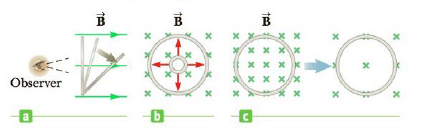Chapter 20, Problem 3CQ

Chapter
Section
Textbook Problem

Figure CQ20.3 shows three views of a circular loop in a magnetic field. In each view, the illustrated change results in an induced current. Indicate the direction of this induced current as either CW (for clockwise) or CCW (for counter clockwise) if: (a) the loop (viewed edge-on) is rotated away from perpendicular to the magnetic field, (b) the loop area increases, and (c) the magnetic field weakens.Figure CQ20.3

(a)

To determine
To find the direction of current induced in the loop

Explanation

According to Lenz’s law whenever the magnetic flux associated with loop changes the emf induces and hence current flows through the coil. And this current flow in such a way that the change in magnetic flux opposes by flowing the current through the loop

The loop is changing angle from perpendicular as a re...

(b)

To determine
To find the direction of current induced in circular loop when area of the loop keep increasing

(c)

To determine
To find the direction of current induced in the circular loop if magnetic field weakens.

Still sussing out bartleby?

Check out a sample textbook solution.

See a sample solution

The Solution to Your Study Problems

Bartleby provides explanations to thousands of textbook problems written by our experts, many with advanced degrees!

Get Started

Find more solutions based on key concepts